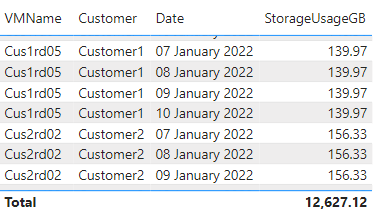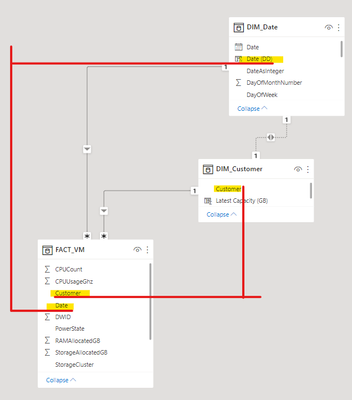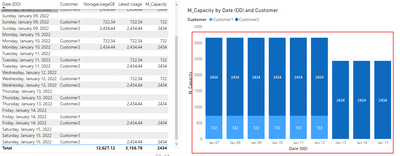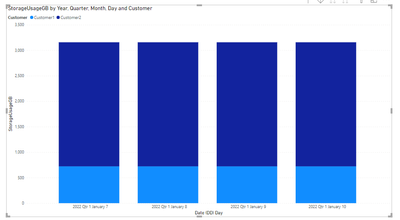cancel
Showing results for
Did you mean:

Fabric is Generally Available. Browse Fabric Presentations. Work towards your Fabric certification with the Cloud Skills Challenge.Helper I

## Fill future dates with latest data and Filter Dates across two tables

Hello,

Hopefully someone can help!

I'm trying to calculate the amount of used storage available in our Vcenter over time, and then forecast this against customer contract termination dates. If anyone could point me in the right direction, I'd be grateful! I've attached the pbix below, and the DAX of my current measure.

``````M_Usage =
VAR _LASTDATE = CALCULATE(MAX(FACT_VM[Date]),ALL(Fact_VM))
VAR _LASTCAPACITY = CALCULATE(SUM(FACT_VM[StorageUsageGB]),FILTER(ALL(Fact_VM),Fact_VM[Date] = _LASTDATE))
VAR _FirstEOLDate = MIN(DIM_Customer[NextTerminationDate])
VAR _FirstEOLHOST = CALCULATE(MAX(DIM_Customer[Customer]),FILTER(ALL(DIM_Customer),DIM_Customer[NextTerminationDate] = _FirstEOLDate))
VAR _CAPACITYAFTEREOL = CALCULATE(SUM(FACT_VM[StorageUsageGB]),FILTER(ALL(Fact_VM),AND(Fact_VM[Date] = _LASTDATE,FACT_VM[VMName]<>_FirstEOLHOST)))
VAR _IF = IF(MAX(DIM_Date[Date])<=_LASTDATE,SUM(FACT_VM[StorageUsageGB]),IF(MAX(DIM_Date[Date])<_FirstEOLDate,_LASTCAPACITY,_CAPACITYAFTEREOL))
VAR _RESULT = IF(_IF = BLANK(),SUM(FACT_VM[StorageUsageGB]),_IF)
RETURN
_RESULT``````

Example/Requirements:

1. Data for both customers is available from the 7th to 10th of January 2022.

2. Data should fill down after the latest data per customer, in this case, after the 10th of January.

3. Customer 1 expires on the 12th, prevent data from Customer 1 from showing after this point.

4. Retain ability to show each customers value per day.

Desired Result:I have 3 tables to accomplish this;

DIM_DateDIM_CustomerFACT_VMRelationship:1 ACCEPTED SOLUTIONSuper User

Something like this?``````M_Capacity =
var d = max(DIM_Date[Date])
var m = CALCULATE(max(FACT_VM[Date]),FACT_VM[Date]<=d)
var s= CALCULATE(sum(FACT_VM[StorageUsageGB]),FACT_VM[Date]=m)
return if(d<SELECTEDVALUE(DIM_Customer[NextTerminationDate]),s)``````See attached.

4 REPLIES 4Community Support

Hi, @AndyB ;

You could try it.

``````M_Capacity =
VAR _LASTDATE = CALCULATE(MAX(FACT_VM[Date]),ALL(Fact_VM))
VAR _LASTCAPACITY = CALCULATE(SUM(FACT_VM[StorageUsageGB]),FILTER(ALL('FACT_VM'),[Customer]=MAX('DIM_Customer'[Customer])&&[Date]=_LASTDATE))
RETURN
IF(MAX('DIM_Date'[Date (DD)])<=MAX('DIM_Customer'[NextTerminationDate])&&MAX('DIM_Customer'[Customer])<>BLANK(),
IF( MAX('DIM_Date'[Date (DD)]) in VALUES('FACT_VM'[Date]),
SUM(FACT_VM[StorageUsageGB]),_LASTCAPACITY))``````

The final output is shown below:Best Regards,
Community Support Team_ Yalan Wu
If this post helps, then please consider Accept it as the solution to help the other members find it more quickly.Super User

Your sample data doesn't seem to match the data from the desired outcome (regardless of expiry)  I see 3TB usage rather than 500GB - can you please clarify?I am also pretty sure that you should not need the inactive relationship. That can be covered by a measure calculation instead.Super User

Something like this?``````M_Capacity =
var d = max(DIM_Date[Date])
var m = CALCULATE(max(FACT_VM[Date]),FACT_VM[Date]<=d)
var s= CALCULATE(sum(FACT_VM[StorageUsageGB]),FACT_VM[Date]=m)
return if(d<SELECTEDVALUE(DIM_Customer[NextTerminationDate]),s)``````See attached.Helper I

Thank you very much @lbendlin  & @v-yalanwu-msft , both of your solutions worked brilliantly! I really appreciate you taking the time to respond and provide the measures, and will now be able to use and adapt these going forward!Announcements#### Power BI Monthly Update - November 2023

Check out the November 2023 Power BI update to learn about new features.#### Fabric Community News unified experience

Read the latest Fabric Community announcements, including updates on Power BI, Synapse, Data Factory and Data Activator.#### The largest Power BI and Fabric virtual conference

130+ sessions, 130+ speakers, Product managers, MVPs, and experts. All about Power BI and Fabric. Attend online or watch the recordings.Top Solution Authors
Top Kudoed Authors
Users online (2,833)